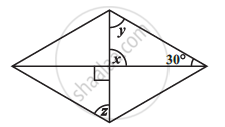Share

# Consider the Given Parallelograms. Find the Values of the Unknowns X, Y, Z. - 2 - CBSE Class 8 - Mathematics

ConceptKinds of Quadrilaterals Concept of Parallelogram

#### Question

Consider the given parallelograms. Find the values of the unknowns x, y, z.#### Solution

x = 90° (Vertically opposite angles)

x + y + 30° = 180° (Angle sum property of triangles)

120° + y = 180°

y = 60°

Is there an error in this question or solution?

#### APPEARS IN

NCERT Solution for Mathematics Textbook for Class 8 (2018 to Current)